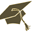Thesis (Selection of subject)(version: 290)
Assignment details
Login via CASGeometrická mechanika kontinua
Thesis title in Czech: Geometrická mechanika kontinua Goemetric continuum mechanics Lie algebra, Hamiltonian mechanics, Poisson bracket, fluid mechanics, solid mechanics, semidirect product 2018/2019 diploma thesis Mathematical Institute of Charles University (32-MUUK) RNDr. Michal Pavelka, Ph.D.
 Guidelines Classical mechanics of point particles can be rewritten as Hamilton canonical equations, which are generated by a Hamiltonian and by the canonical Poisson bracket. This is the simplest case of Hamiltonian mechanics. In the case of continuum (or fields), for instance electromagnetism, kinetic theory, fluid mechanics, non-Newtonian fluid mechanics and solid mechanics can be also seen as being generated by a Poisson bracket and energy, and they are thus infinite-dimensional Hamiltonian systems. Hamiltonian mechanics is a geometrical approach unifying reversible evolution in continuum mechanics. We shall study the following points: 1) Review the construction of fluid mechanics as a Lie-Poisson Hamiltonian system including entropy [1,2,3]. 2) Construct the mechanics of distortion matrix (unifying the description of fluids, solids and non-Newtonian fluids) geometrically (semidirect product). 3) Review the possibility of Hamiltonian numerical schemes for continuum mechanics .
 References  V.I. Arnold. Sur la géometrie différentielle des groupes de Lie de dimension infini et ses applications dans l’hydrodynamique des fluides parfaits. Annales de l’institut Fourier, 16(1):319–361, 1966.  J Marsden, T Ratiu, and A Weinstein. Semidirect products and reduction in mechanics. Transactions of the american mathematical society, 281(1):147–177, 1984. ISSN 0002-9947. doi: 10.2307/1999527.  Michal Pavelka, Václav Klika, and Miroslav Grmela. Multiscale Thermo-Dynamics. de Gruyter (Berlin), 2018.  E. S. Gawlik, P. Mullen, D. Pavlov, J. E. Marsden, M. Desbrun, Geometric, Variational Discretization of Continuum Theories, Physica D: Nonlinear Phenomena (240(21), pp. 1724-1760, 2011)

Charles University | Information system of Charles University | http://www.cuni.cz/UKEN-329.html